# Three Phase Line Commutated Converter - 2 Notes | Study Power Electronics - Electrical Engineering (EE)

## Electrical Engineering (EE): Three Phase Line Commutated Converter - 2 Notes | Study Power Electronics - Electrical Engineering (EE)

The document Three Phase Line Commutated Converter - 2 Notes | Study Power Electronics - Electrical Engineering (EE) is a part of the Electrical Engineering (EE) Course Power Electronics.
All you need of Electrical Engineering (EE) at this link: Electrical Engineering (EE)

Higher pulse number converters and dual converter

The three phase fully controlled converter is widely used in the medium to moderately high power applications. However in very large power applications (such as HV DC transmission systems) the device ratings become impractically large. Also the relatively low frequency (6th in the dc side, 5th and 7th in the ac side) harmonic voltages and currents produced by this converter become unacceptable. Therefore several such converters are connected in series parallel combination in order to increase the voltage / current rating of the resulting converter. Furthermore if the component converters are controlled properly some lower order harmonics can be eliminated both from the input and output resulting in a higher pulse converter.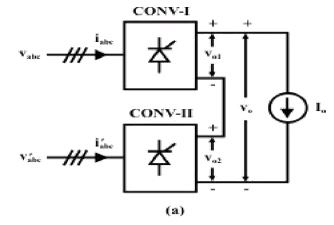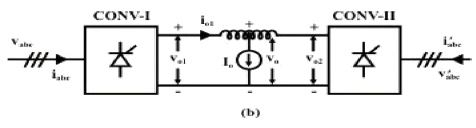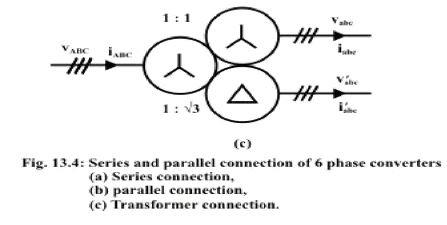Fig. 13.4(a) schematically represents series connection of two six pulse converters where as Fig. 13.4(b) can be considered to be a parallel connection. The inductance in between the converters has been included to limit circulating harmonic current. In both these figures CONV – I and CONV – II have identical construction and are also fired at the same firing angle α. Their input supplies also have same magnitude but displaced in phase by an angle φ. Then one can write

Now if cos 3Kφ = 0 for some K then the corresponding harmonic disappear from the fourier series expression of v0.

In particular if φ = 30° then cos 3Kφ = 0 for K = 1, 2, 3, 5…….
This phase difference can be obtained by the arrangement shown in Fig. 13.4(c).
Then

It can be seen that the frequency of the harmonics present in the output voltage has the form 12ω, 24ω, 36ω ………..
Similarly it can be shown that the input side line current iABC have harmonic frequency of the form
11ω, 13ω, 23ω, 25ω, 35ω, 37ω, ………….
Which is the characteristic of a 12 pulse converter.
In a similar manner more number of 3 phase 6 pulse converters can be connected in series / parallel and the φ angle can be adjusted to obtain 18 and 24 pulse converters.

One of the shortcomings of a three phase fully controlled converter is that although it can produce both positive and negative voltage it can not supply current in both directions. However, some applications such as a four quadrant dc motor drive require this capability from the dc source. This problem is easily mitigated by connecting another three phase fully controlled converter in anti parallel as shown in Fig. 13.5 (a). In this figure converter -I supplies positive load current while converter-II supplies negative load current. In other words converter-I operates in the first and fourth quadrant of the output v – i plane whereas converter-II operates in the third and fourth quadrant. Thus the two converters taken together can operate in all four quadrants and is capable of supplying a four quadrant dc motor drive. The combined converter is called the Dual converter.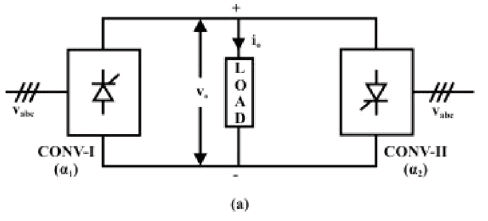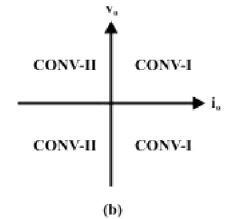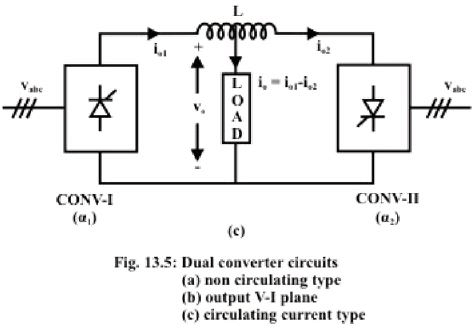Obviously since converter-I and converter-II are connected in antiparallel they must produce the same dc voltage. This requires that the firing angles of these two converters be related as
α2 = π – α1              (13.30)

Although Equations 13.30 ensures that the dc voltages produced by these converters are equal the output voltages do not match on an instantaneous basis. Therefore to avoid a direct short circuit between two different supply lines the two converters must never be gated simultaneously. Converter-I receives gate pulses when the load current is positive. Gate pulses to converter-II are blocked at that time. For negative load current converter-II thyristors are fired while converter-I gate pulses are blocked. Thus there is no circulating current flowing through the converters and therefore it is called the non-circulating current type dual converter. It requires precise sensing of the zero crossing of the output current which may pose a problem particularly at light load due to possible discontinuous conduction. To overcome this problem an interphase reactor may be incorporated between the two converters. With the interphase reactor in place both the converters can be gated simultaneously with α2 = π – α1. The resulting converter is called the circulating current type dual converter.

Gate Drive circuit for three phase fully controlled converter

Several schemes exist to generate gate drive pulses for single phase or three phase converters. In many application it is required that the output of the converter be proportional to a control voltage. This can be achieved as follows.

The following circuit can be used to generate “α” according to equation 13.32.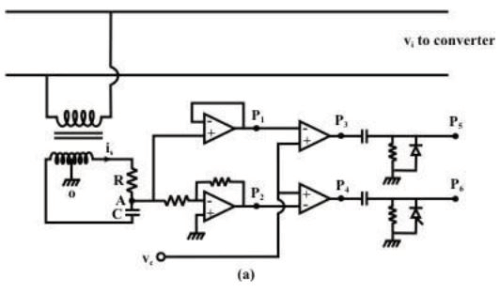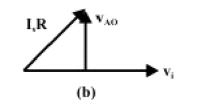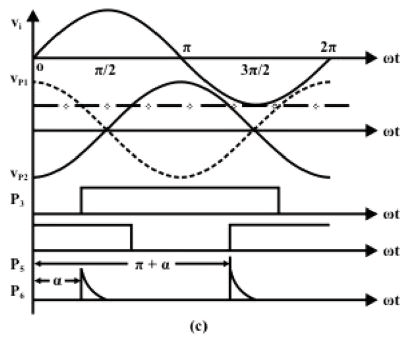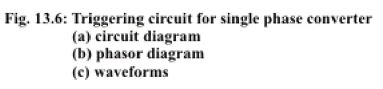In the circuit of Fig. 13.6(a) a phase shift network is used to obtain a waveform leading vi by 90º. The phasor diagram of the phase shift circuit is shown in Fig. 13.6(b). The output of the phase shift waveform (and its inverse) is compared with vc. The firing pulse is generated at the point when these two waveforms are equal. Obviously at-this instant

Therefore this method of generation of converter firing pulses is called “inverse cosine” control. The output of the phase shift network is called carrier waveform.

Similar technique can be used for three phase converters. However the phase shift network here consists of a three phase signal transformer with special connections as shown in Fig. 13.7.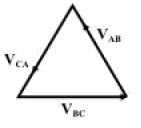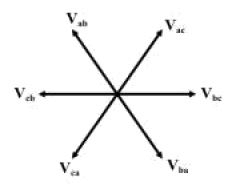The signal transformer uses three single phase transformer each of which has two secondary windings. The primary windings are connected in delta while the secondary windings are connected in zigzag. From Fig. 13.1 (c) T2 is fired α angle after the positive going zero crossing of vbc. Therefore, to implement inverse cosine the carrier wave for Tmust lead vbc by 90º. This waveform is obtained from zigzag connection of the winding segments a1aand c1c 2 as shown in Fig. 13.7(a). The same figure also shows the zigzag connection for other phase. The voltage across each zigzag phase can be used to fire two thyristors belonging to the same phase leg using a circuit similar to Fig. 13.6 (a). The phase shift network will not be required in this case.

Exercise 5.3

1. Fill in the blank(s) with the appropriate word(s)

i) Higher pulse number converters can be realized by __________ and _______ connection of six pulse converters.

ii) Constituent six pulse converters of a 12 pulse converter have _________ firing angles.

iii) The input supply voltages to the converters of a 12 pulse converter have ________magnitudes and are phase shifted from one another by _________ degrees.

iv) The input supply to a 12 pulse converter can be obtained through a _________ connected transformer.

v) Dual converters are used for supplying ________ quadrant dc motor drives.

vi) In a dual converter if one converter is fired at an angle ‘α’ the other has to be fired at _________.

vii) In ___________ current dual converter only one converter conducts t any time.

viii) In a circulating current type dual converter an __________ is used between the converters to limit the circulating current.

ix) To obtain a linear control relation between the control voltage and the output dc voltage of a converter ___________ control logic is used.

x) In a three phase fully controlled converter the carrier waves for firing pulse generation are obtained using three ___________ connected single phase transformers.

Answers: (i) Series, parallel; (ii) same, (iii) equal, 30, (iv) star – star – delta; (v) four; (vi) π - α, (vii) non-circulating ; (viii) inductor, (ix) inverse-cosine; (x) delta-zigzag.

3. What will happen if the signal transformers generating the carrier wave have delta – double star connection instead of delta-zigzag connection.

With delta-double star connection of the signal transformers the carrier wave forms will be in phase with the line voltage waveforms. Therefore, without a phase shift network it will not be possible to generate carrier waveforms which are in quadrature with the line voltages. Hence inverse casine control law cannot be implemented.

Summary

• A three phase fully controlled converter is realized by replacing the diodes of an uncontrolled converter with thyristors.
• A three phase fully controlled converter can operate either as a rectifier or as an inverter.
• The output voltage of a three phase fully controlled converter contains multiple of sixth harmonic of the input frequency in addition to the dc component.
• The input current of a three phase fully controlled converter contains only odd harmonics other than tripler harmonics.
• The input current displacement factor of a three phase fully controlled converter is cos α. α being the firing angle.
• In the continuous conduction mode a three phase fully controlled converter may operate in the inverting mode by increasing α beyond 90º.
• In the inverting mode the firing angle should be less than 180º for safe commutation of the thyristors.
• Several units of three phase fully controlled converters can be connected in series parallel to form higher pulse number (12, 18, 24 etc) converters.
• In higher pulse number converters all component converters are fired at the same firing angle while their input supplies are phase shifted from one another by a predetermined angle.
• Two three phase fully controlled converter can be connected in anti parallel to form a dual converter which can operate in all four quadrants of the V-I plane.
• Dual converters can be of circulating and non circulating current type.
• Fully controlled converters employ “inverse casine control” strategy for generating firing pulses which gives linear relationship between the output voltage and the control voltage. In a three phase fully controlled converter, a three phase delta/zig-zag connected signal transformer is used to generate the required carrier waves for this purpose.
The document Three Phase Line Commutated Converter - 2 Notes | Study Power Electronics - Electrical Engineering (EE) is a part of the Electrical Engineering (EE) Course Power Electronics.
All you need of Electrical Engineering (EE) at this link: Electrical Engineering (EE)Use Code STAYHOME200 and get INR 200 additional OFF

## Power Electronics

5 videos|13 docs|49 tests

Track your progress, build streaks, highlight & save important lessons and more!

,

,

,

,

,

,

,

,

,

,

,

,

,

,

,

,

,

,

,

,

,

;